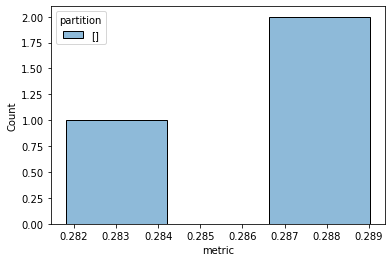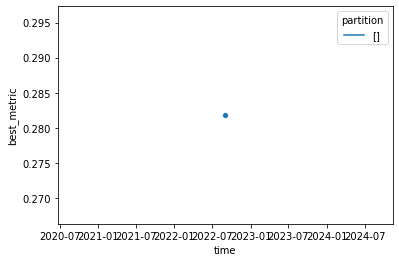# Non-Iterative Problems#

Non-iterative problems converge to an optimum solution as opposed to iterative problems that don’t have a definite end of tuning. Scikit-learn models generally fall under this. In this section we see how to work with these types of problems using the Space class we learned in the last section.

Have questions? Chat with us on Github or Slack:

## Setup#

We will use the California Housing dataset and train scikit-learn models on it. This code snippet will show how to load the data, but in practice it should be loaded in the objective function as seen below. This will make the code more scalable when running on a distributed backend like Spark or Dask. The cluster workers will be able to directly load the data rather than have it pass through the scheduler.

import pandas as pd
from sklearn.datasets import fetch_california_housing

# get training data
def get_data():
return fetch_california_housing(as_frame=True).frame


MedInc HouseAge AveRooms AveBedrms Population AveOccup Latitude Longitude MedHouseVal
0 8.3252 41.0 6.984127 1.023810 322.0 2.555556 37.88 -122.23 4.526
1 8.3014 21.0 6.238137 0.971880 2401.0 2.109842 37.86 -122.22 3.585
2 7.2574 52.0 8.288136 1.073446 496.0 2.802260 37.85 -122.24 3.521
3 5.6431 52.0 5.817352 1.073059 558.0 2.547945 37.85 -122.25 3.413
4 3.8462 52.0 6.281853 1.081081 565.0 2.181467 37.85 -122.25 3.422

## Objective Function for Non-Iterative Problems#

Tune requires that the objective function for non-iterative problems return either a float or Tuple[float, Dict[str, Any]]. The latter one is used to return both the metric and the metadata. It is required to have the type annotations so that Tune knows the structure of the objective function. For more involved objective functions where the properties of a trial are needed, users can use the NonIterativeObjectiveFunc class to define their objective function as seen later in the tutorials. This is just the preferred and most minimal approach to write out the objective function.

def objective1(a, b) -> float:
return a**2 + b**2

def objective2(a, b) -> Tuple[float, Dict[str, Any]]:


For this example, our objective function to take in a model and the accompanying hyperparameters for the model. We then return the mean_absolute_percentage_error across the folds of model training with cross validation.

from typing import Tuple, Dict, Any
from sklearn.model_selection import cross_val_score
from sklearn.metrics import mean_absolute_percentage_error, make_scorer

def objective(model:Any, **hp:Any) -> float:
model_ins = model(**hp)
data = get_data()
y = data["MedHouseVal"]
x = data.drop("MedHouseVal", axis=1)
scores = cross_val_score(model_ins, x, y, cv=3,
scoring=make_scorer(mean_absolute_percentage_error))
return scores.mean()


We can test this objective function by passing in sample models already even without the Tune library. These will give us benchmark values.

from lightgbm import LGBMRegressor
from xgboost import XGBRegressor

print("XGB Baseline:", objective(XGBRegressor))
print("LGBM Baseline", objective(LGBMRegressor))

XGB Baseline: 0.28180971125916277
LGBM Baseline 0.26024866093016924


## Constructing the Search Space#

In the previous section of the tutorial, we learned about the Space class and how to construct a search space. Below is an example how to use it for XGBoost and LightGBM. Recall that the Space is decoupled from any specific tuning framework.

from tune import Space, RandInt, Grid

xgb_space = Space(model=XGBRegressor, n_estimators=Grid(100, 200, 300))
lgbm_space = Space(model=LGBMRegressor, n_estimators=RandInt(100, 300))
list(xgb_space + lgbm_space)

[{'model': <class 'xgboost.sklearn.XGBRegressor'>, 'n_estimators': 100},
{'model': <class 'xgboost.sklearn.XGBRegressor'>, 'n_estimators': 200},
{'model': <class 'xgboost.sklearn.XGBRegressor'>, 'n_estimators': 300},
{'model': <class 'lightgbm.sklearn.LGBMRegressor'>, 'n_estimators': RandInt(low=100, high=300, q=1, log=False, include_high=True)}]


## Tuning Non-Iterative Objective Functions#

Now we can use the suggest_for_non_iterative_objective() function to put the objective function and search space together. This function has a lot more functionality, this is just the basic use case. It will return the best result among the trials.

Because we have a parameter called model in the Space, this will be fed into the objective function model argument.

from tune import suggest_for_noniterative_objective

result = suggest_for_noniterative_objective(
objective,
xgb_space,
)
result

NativeExecutionEngine doesn't respect num_partitions ROWCOUNT

[{'trial': {'trial_id': 'fcfcdf23-1a9f-56a2-8638-6a32280d8ed0', 'params': {'model': <class 'xgboost.sklearn.XGBRegressor'>, 'n_estimators': 100}, 'metadata': {}, 'keys': []}, 'metric': 0.28180971125916277, 'params': {'model': <class 'xgboost.sklearn.XGBRegressor'>, 'n_estimators': 100}, 'metadata': {}, 'cost': 1.0, 'rung': 0, 'sort_metric': 0.28180971125916277, 'log_time': datetime.datetime(2022, 9, 1, 0, 49, 1, 587778)}]


The result contains the metrics and metadata of the best trial.

## Tuning with an Optimizer#

The example above only used the xgb_space because the lgbm_space has a stochastic expression RandInt. Stochastic expressions need to be paired with a local_optimizer such as Optuna or Hyperopt because the value of that parameter needs to be tuned. In the example below, we attach hyperopt:5 which means that we’ll use Hyperopt with a maximum of 5 iterations.

result = suggest_for_noniterative_objective(
objective,
xgb_space + lgbm_space,
local_optimizer = "hyperopt:5",
)
result

NativeExecutionEngine doesn't respect num_partitions ROWCOUNT

[{'trial': {'trial_id': 'c1c398bb-ef8f-5a95-acdf-e4b190cd1696', 'params': {'model': <class 'lightgbm.sklearn.LGBMRegressor'>, 'n_estimators': 199}, 'metadata': {}, 'keys': []}, 'metric': 0.25905132621704874, 'params': {'model': <class 'lightgbm.sklearn.LGBMRegressor'>, 'n_estimators': 199}, 'metadata': {}, 'cost': 1.0, 'rung': 0, 'sort_metric': 0.25905132621704874, 'log_time': datetime.datetime(2022, 9, 1, 0, 49, 20, 507151)}]


## Returning Top N Trials#

By default, Tune only returns the best trial. To return more, use the top_n parameter.

result = suggest_for_noniterative_objective(
objective,
xgb_space + lgbm_space,
local_optimizer = "hyperopt:5",
top_n=3
)
result

NativeExecutionEngine doesn't respect num_partitions ROWCOUNT

[{'trial': {'trial_id': 'c1c398bb-ef8f-5a95-acdf-e4b190cd1696', 'params': {'model': <class 'lightgbm.sklearn.LGBMRegressor'>, 'n_estimators': 199}, 'metadata': {}, 'keys': []}, 'metric': 0.25905132621704874, 'params': {'model': <class 'lightgbm.sklearn.LGBMRegressor'>, 'n_estimators': 199}, 'metadata': {}, 'cost': 1.0, 'rung': 0, 'sort_metric': 0.25905132621704874, 'log_time': datetime.datetime(2022, 9, 1, 0, 49, 33, 298827)},
{'trial': {'trial_id': 'fcfcdf23-1a9f-56a2-8638-6a32280d8ed0', 'params': {'model': <class 'xgboost.sklearn.XGBRegressor'>, 'n_estimators': 100}, 'metadata': {}, 'keys': []}, 'metric': 0.28180971125916277, 'params': {'model': <class 'xgboost.sklearn.XGBRegressor'>, 'n_estimators': 100}, 'metadata': {}, 'cost': 1.0, 'rung': 0, 'sort_metric': 0.28180971125916277, 'log_time': datetime.datetime(2022, 9, 1, 0, 49, 28, 714342)},
{'trial': {'trial_id': 'e130a880-2ba8-522e-adc5-58bd9b4bc542', 'params': {'model': <class 'xgboost.sklearn.XGBRegressor'>, 'n_estimators': 200}, 'metadata': {}, 'keys': []}, 'metric': 0.2869639289729983, 'params': {'model': <class 'xgboost.sklearn.XGBRegressor'>, 'n_estimators': 200}, 'metadata': {}, 'cost': 1.0, 'rung': 0, 'sort_metric': 0.2869639289729983, 'log_time': datetime.datetime(2022, 9, 1, 0, 49, 32, 368481)}]


## Running Distributedly#

Because Tune is built on top of the Fugue project, the hyperparameter tuning can be run distributedly on a cluster on all of the backends that Fugue supports (Spark, Dask, Ray). In order to do this, all we need to do is specify the execution_engine. In this case, we can pass in a SparkSession. To use Dask, we can supply a Dask Client to the execution_engine.

from pyspark.sql import SparkSession
spark = SparkSession.builder.getOrCreate()

result = suggest_for_noniterative_objective(
objective,
xgb_space + lgbm_space,
local_optimizer = "hyperopt",
execution_engine=spark
)
result



[{'trial': {'trial_id': 'c1c398bb-ef8f-5a95-acdf-e4b190cd1696', 'params': {'model': <class 'lightgbm.sklearn.LGBMRegressor'>, 'n_estimators': 113}, 'metadata': {}, 'keys': []}, 'metric': 0.2587359331529883, 'params': {'model': <class 'lightgbm.sklearn.LGBMRegressor'>, 'n_estimators': 113}, 'metadata': {}, 'cost': 1.0, 'rung': 0, 'sort_metric': 0.2587359331529883, 'log_time': datetime.datetime(2022, 9, 1, 0, 52, 57, 29)}]


For local parallelization on Spark and Dask, gradient boosting based models try to occupy as many cores on the machine as possible so there will not be any speedup to using Spark or Dask.

## Real-Time Monitoring#

For real time monitoring in Jupyter notebooks, a monitor argument can be passed. There are some built-in monitors. In the example below, we use the "hist" monitor which updates a histogram of the metric value. These are interactive plots that update inside a Jupyter notebook run. Because this documentation is already rendered, only the final image is displayed.

result = suggest_for_noniterative_objective(
objective,
xgb_space,
monitor="hist"
)[] 0.28180971125916277 {'trial': {'trial_id': 'fcfcdf23-1a9f-56a2-8638-6a32280d8ed0', 'params': {'model': <class 'xgboost.sklearn.XGBRegressor'>, 'n_estimators': 100}, 'metadata': {}, 'keys': []}, 'metric': 0.28180971125916277, 'params': {'model': <class 'xgboost.sklearn.XGBRegressor'>, 'n_estimators': 100}, 'metadata': {}, 'cost': 1.0, 'rung': 0, 'sort_metric': 0.28180971125916277, 'log_time': datetime.datetime(2022, 9, 1, 1, 4, 13, 613623)}


This can also be used with Spark or Dask as long as we specify an RPCClient. The RPCClient also comes from Fugue. In the example below, we use "ts" to monitor the up-to-date best metric.

callback_conf = {
}

result = suggest_for_noniterative_objective(
objective,
xgb_space,
execution_engine=spark,
execution_engine_conf=callback_conf,
monitor="ts"
)[] 0.28180971125916277 {'trial': {'trial_id': 'fcfcdf23-1a9f-56a2-8638-6a32280d8ed0', 'params': {'model': <class 'xgboost.sklearn.XGBRegressor'>, 'n_estimators': 100}, 'metadata': {}, 'keys': []}, 'metric': 0.28180971125916277, 'params': {'model': <class 'xgboost.sklearn.XGBRegressor'>, 'n_estimators': 100}, 'metadata': {}, 'cost': 1.0, 'rung': 0, 'sort_metric': 0.28180971125916277, 'log_time': datetime.datetime(2022, 9, 1, 1, 12, 6, 306297)}


## Logging Tuning Results to Experiment Trackers#

It is common to pair Tune with an experiment tracker like MLFlow or Weights and Biases. These platforms will track the previous runs and persist artifacts related to model training. In the example below, we use Weights and Biases and pass a project name. Because this needs an account and API Key to run, we can’t run the next cell.

result = suggest_for_noniterative_objective(
objective,
xgb_space + lgbm_space,
local_optimizer = "hyperopt:5",
execution_engine = client,
logger = "wandb:CA_housing_tuning"
)


## Tuning per Partition#

For big data, we may want to split the data into multiple partitions and tune the search space for each individual group. We don’t have any good categorical column in the California Housing dataset, so let’s change the HouseAge column to a categorical. We will change it to low and high.

data = get_data()
data = data.assign(HouseAge=pd.qcut(data["HouseAge"], q = [0, 0.5, 1], labels=['low','high']))

MedInc HouseAge AveRooms AveBedrms Population AveOccup Latitude Longitude MedHouseVal
0 8.3252 high 6.984127 1.023810 322.0 2.555556 37.88 -122.23 4.526
1 8.3014 low 6.238137 0.971880 2401.0 2.109842 37.86 -122.22 3.585
2 7.2574 high 8.288136 1.073446 496.0 2.802260 37.85 -122.24 3.521
3 5.6431 high 5.817352 1.073059 558.0 2.547945 37.85 -122.25 3.413
4 3.8462 high 6.281853 1.081081 565.0 2.181467 37.85 -122.25 3.422

We can make this into a Spark DataFrame that will then be partitioned. Training per partition will also work on Pandas DataFrames.

spark_data = spark.createDataFrame(data)


The objective function needs to change a bit because we previously loaded the data inside. Instead, we will pass in the partition as a Pandas DAtaFrame.

def new_objective(data: pd.DataFrame, model:Any, **hp:Any) -> float:
# data is already partitioned by House Age when it comes in
model_ins = model(**hp)
model_age = data.iloc["HouseAge"]
y = data["MedHouseVal"]
x = data.drop(["MedHouseVal", "HouseAge"], axis=1)
scores = cross_val_score(model_ins, x, y, cv=3,
scoring=make_scorer(mean_absolute_percentage_error))
return scores.mean()


Below is an example of how to put everything together in this section. We use a Spark DataFrame that is partitioned into multiple Pandas DataFrame, and we tune the Space for each partition. In order to do this, we need to supply the DataFrame as df ahead of time along with the partition_keys. The temp_path is needed to store temporary files.

result = suggest_for_noniterative_objective(
new_objective,
xgb_space + lgbm_space,
local_optimizer="hyperopt",
execution_engine=spark,
df = spark_data,
df_name = "data",
temp_path="/tmp/",
partition_keys=["HouseAge"]
)
result



[{'trial': {'trial_id': '81cd2484-994f-5704-800f-23b791d8d893', 'params': {'model': <class 'lightgbm.sklearn.LGBMRegressor'>, 'n_estimators': 124}, 'metadata': {}, 'keys': ['high']}, 'metric': 0.2713623100846554, 'params': {'model': <class 'lightgbm.sklearn.LGBMRegressor'>, 'n_estimators': 124}, 'metadata': {}, 'cost': 1.0, 'rung': 0, 'sort_metric': 0.2713623100846554, 'log_time': datetime.datetime(2022, 9, 1, 1, 38, 56, 71338)},
{'trial': {'trial_id': '849f6270-6e81-5ba8-b2c2-ec9ba0718289', 'params': {'model': <class 'lightgbm.sklearn.LGBMRegressor'>, 'n_estimators': 127}, 'metadata': {}, 'keys': ['low']}, 'metric': 0.27277650051184715, 'params': {'model': <class 'lightgbm.sklearn.LGBMRegressor'>, 'n_estimators': 127}, 'metadata': {}, 'cost': 1.0, 'rung': 0, 'sort_metric': 0.27277650051184715, 'log_time': datetime.datetime(2022, 9, 1, 1, 39, 10, 137673)}]


## Conclusion#

In this section, we went over the features of the suggest_for_noniterative_objective. We showed how the Space and objective function can be defined without worrying about the underlying optimization framework. When ready to perform tuning, we can bring them together and specify the local_optimizer and execution_engine to run our jobs on. Because Tune is built on top of Fugue, we can run Hyperopt or Optuna on top of Spark, Dask, and Ray.

We can also utilize distributed compute to tune the search space for each partition and get the best results. All we had to do was supply partition_keys to our Spark DataFrame and we were able to find the best model for each group of data.

Tune lets users define their tuning problems in a minimal grammar, and then takes care of executing it on top of specifed optimizers and engines.